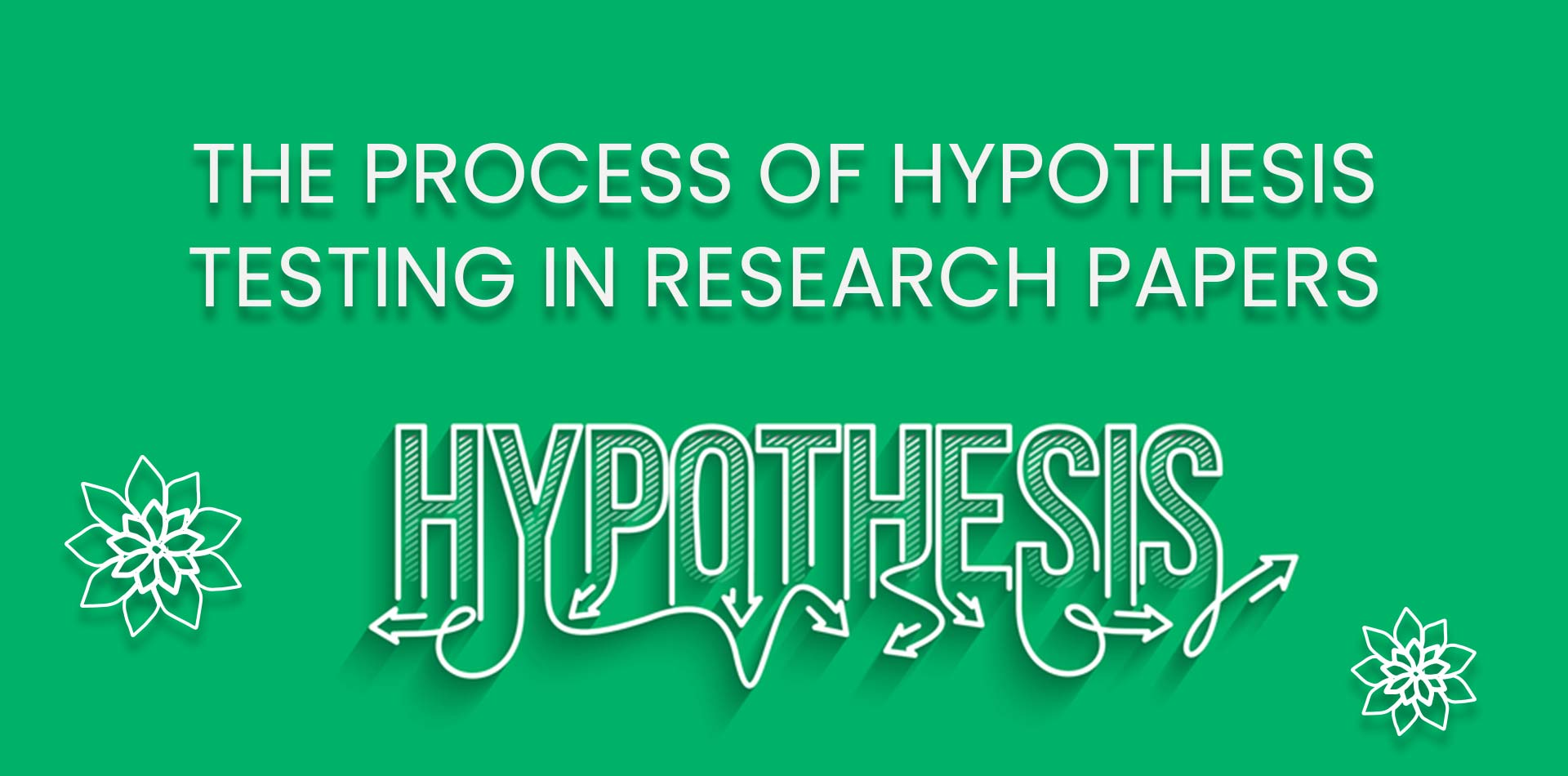# Process of hypothesis testing in research papers

Total Views: 561Daily Views: 1
Categories: Education

Process of hypothesis testing in research papers Hypothesis testing is a statistical method used to make inferences about a population based on a sample of data. It is a common technique used in research papers to test the validity of a research hypothesis. The process of hypothesis testing involves several steps, including formulating a null hypothesis and an alternative hypothesis, selecting a sample, determining a test statistic, and making a decision about the null hypothesis. In this article, learn the Process of hypothesis testing in research papers.

• ## Formulating a null hypothesis and an alternative hypothesis

The first step in hypothesis testing is to formulate a null hypothesis and an alternative hypothesis. The null hypothesis (H0) is the statement that there is no significant difference or relationship between the variables being studied. The alternative hypothesis (Ha) is the statement that there is a significant difference or relationship between the variables being studied. The researcher must choose one of these hypotheses to test, and the decision about which hypothesis to test depends on the research question and the research design.

• ### Selecting a simple

Once the hypotheses have been formulated, the next step is to select a sample. The sample should be representative of the population and should be selected using a random sampling method. The sample size is also an important consideration, as a larger sample size will increase the power of the test and decrease the chance of a type II error.

• ### Determining a test statistic

After the sample has been selected, the researcher must determine a test statistic. A test statistic is a numerical value that is calculated based on the sample data and is used to evaluate the null hypothesis. Common test statistics include the t-test, chi-square, and F-test, among others. The choice of test statistic depends on the type of data, the sample size, and the research design.

• ### Making a decision about the null hypothesis

Once the test statistic has been calculated, the researcher must make a decision about the null hypothesis. This decision is based on the calculated test statistic and a pre-determined level of significance (alpha). The level of significance is a probability value that represents the chance of obtaining a test statistic as extreme or more extreme than the one calculated under the assumption that the null hypothesis is true. If the calculated test statistic is greater than the critical value, the null hypothesis is rejected, and the alternative hypothesis is accepted. If the calculated test statistic is less than the critical value, the null hypothesis is not rejected, and the researcher cannot conclude that there is a significant difference or relationship between the variables being studied.

• #### Interpreting the results

The final step in the process of hypothesis testing is to interpret the results. If the null hypothesis is rejected, the researcher can conclude that there is a significant difference or relationship between the variables being studied. If the null hypothesis is not rejected, the researcher cannot conclude that there is a significant difference or relationship between the variables being studied. The researcher should also consider the practical significance of the results in addition to the statistical significance.

It’s important to keep in mind that hypothesis testing is a probabilistic method, meaning that there is a chance of making a type I error (rejecting a true null hypothesis) or a type II error (failing to reject a false null hypothesis). The level of significance (alpha) is used to control the probability of making a type I error, while the sample size and the power of the test are used to control the probability of making a type II error. Additionally, the p-value is also an important part of the process; it is the probability of obtaining a test statistic as extreme or more extreme than the one calculated under the assumption that the null hypothesis is true if the p-value is less than the level of significance, the null hypothesis is rejected.

## Conclusion

In conclusion, hypothesis testing is a powerful tool that researchers use to make inferences about populations based on a sample of data. It is a crucial step in many research papers, as it allows researchers to test the validity of their hypotheses and draw meaningful conclusions about their findings. The process of hypothesis testing involves several steps, including formulating a null hypothesis and an alternative hypothesis, selecting a sample, determining a test statistic, and making a decision about the null hypothesis. It is important to keep in mind that hypothesis testing is a probabilistic method, and there is always a chance of making a type I or type II error. Additionally, it’s important to consider the practical significance of the results in addition to the statistical significance. Overall, understanding and applying the process of hypothesis testing can help researchers to conduct rigorous and meaningful research, and make valuable contributions to the field.